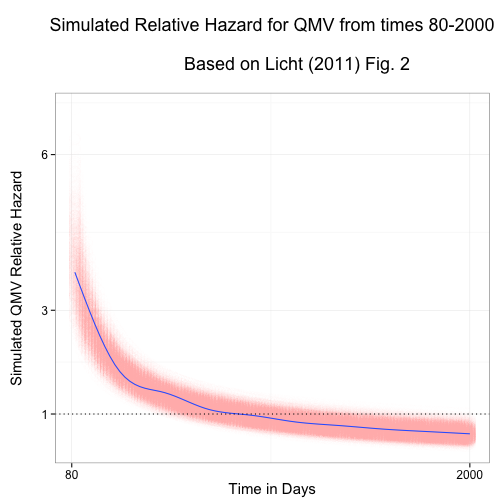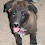### Graphing Non-Proportional Hazards in R

Update 30 July 2013: I've moved all of the functionality described in this post into an R package called simPH. Have a look. It is much easier to use.

Update 30 December 2012: I updated the code HERE so that it keeps only the middle 95 percent of the simulated values.

I really like this article by Amanda Licht in Political Analysis. It gives a lot of information on how to interpret nonproportional hazards and includes some nice graphs.

Her source code is really helpful for learning the nuts and bolts of how to simulate quantities of interests over time. However, it's in Stata code, which doesn't really fit into my R-based workflow at the moment. So I decided to port the code over. This post gives an example of what I did.

## What is a non-proportional hazard & why use them?

Here is my motivation for being interested in non-proportional hazards: In a few papers I used Cox Proportional Hazard (PH) models to examine countries' policy adoption decisions. The problem with Cox PH models is that they assume the risk of adopting a policy at each point in time changes proportionally. However, in my papers I had good reasons to suspect that this risk actually changed non-proportionally over time. To overcome these model-assumption-busting problems I included time interactions, a pretty common way of dealing with the problem.

When you include time interactions, the variables' coefficients ($$\beta_{cc}$$) are now a combination of the estimates for the base coeffiecient ($$\beta_{1}$$) plus the time interaction ($$\beta_{2}(t)$$):

$\beta_{cc} = \beta_{1} + \beta_{2}(t)$

You'll probably notice that the combined coefficient is different at each point in time $$t$$.

See Amanda's original article for a more detailed discussion of these issues.

## The Problem

The problem I had before was conveying the substantive effect of the combined coefficient and our uncertainty about it over time. Licht's article solves these problems with Stata. How do we do it in R?

## Non-proportional Relative Hazards in R

To demonstrate how to achieve these goals I'll recreate part of a figure from Licht's paper using R. This figure uses data/methods from an earlier paper by Golub & Steunenberg (2007). You can download the data here (it's called GolubEUPdata.tab). My goal is a figure plotting simulated values of the relative non-proportional hazard for the variable qmv between times 80 and 2000.

So, this is the figure I created:The full R script for doing this is HERE.

## Step-by-step

These are the main steps I used to create the figure:

• First, run the Cox PH model with time interactions using the coxph command from the survival package. Assign the output of this model to a new object (in my example I call the output object M1).

• Extract the coefficeient and variance-covariance matrices from the output object like this:

# Create coefficient matrix
Coef <- matrix(M1$coefficients) # Create variance-covariance matrix VC <- vcov(M1)  • Using the rmultnorm command from the MSBVAR package, simulate coefficients. Drawn <- rmultnorm(n = 1000, mu = Coef, vmat = VC)  • Clean up the data. For this example we only need two of the columns of simulated coefficients (numbers 1 & 13). We also need to create a simulation ID variable which will be important later. # Keep qmv and Lqmv Drawn <- data.frame(Drawn[, c(1, 13)]) # Create Merge Variable (Simulation Number) Drawn$ID <- 1:1000

• Now that we have the simulated coefficients, we can calculate the simulated combined coefficient for qmv. This involves first creating a vector with the times over which we want to calculate the coefficients. We then combine this with the simulated data as in the equation above.
# Create Combined time interactionsCoefficient
TVSim <- outer(Drawn[,2], Range)
TVSim <- data.frame(melt(TVSim))
TVSim <- rename(TVSim, c(X1 = "ID", X2 = "Time", value = "TVR"))

# Merge in the non-time interacted coefficient and combine
TVSim <- merge(Drawn, TVSim, by = "ID")
TVSim$CCqmv <- TVSim$qmv + TVSim$TVR  • Now we can exponentiate the coefficient to get the relative hazard # Create Combined Relative Hazard TVSim$HRqmv <- exp(TVSim$CCqmv)  • Finally, after ordering the data by time, we can plot our simulated relative hazards over time. # Order Variables TVSim <- TVSim[order(TVSim$Time),]

# Graph Simulated Combined Hazard Ratios
ggplot(TVSim, aes(Time, HRqmv)) +
geom_point(shape = 21, alpha = I(0.01), colour = "#FA9FB5", size = 5) +
geom_smooth() +
geom_hline(aes(yintercept = 1), linetype = "dotted") +
scale_y_continuous(breaks = c(-1, 0, 1, 3, 6)) +
scale_x_continuous(breaks = c(0, 129), labels = c(80, 2000)) +
xlab("Time in Days") + ylab("Simulated QMV Relative Hazard\n") +
ggtitle("Simulated Relative Hazard for QMV from times 80-2000\n
Based on Licht (2011) Fig. 2\n") +
theme_bw(base_size = 15)


Licht points out that the relative hazard is substantively interesting for dummy variables (which have values 0 and 1), but we probably want other approaches for continuous variables. Only a few modifications to the code above are required to implement these.

Thanks to Jeff Chweiroth for pointing me to Amanda Licht's article and motivating me to put together this code.Ása Johannesen said…
Hi!

I'm really quite useless at this (I wish I could say it's because I'm new at it), but as I'm struggling with nonproportionality and stumbled across you while googling, I thought I might ask for your input.

I'd love to include interaction terms with time for one of my factors, but the model won't converge if I do that. Is it because this sort of interaction only works for continuous (or otherwise) covariates and not with categorical factors? Would it help to create binary 0/1 dummy factors? I'm actually quite interested in how the effects of my factors change over time and stratification is awkward as I need main effects from all (two) factors.

Also, why is it so common to use log time?Unknown said…
Hi Asa

In theory you should be able to include categorical factors with many variables. Like you mention, I think you would probably need a separate TVC for each factor level. This might get a little hard to handle, but without your data and models I can't really give much advice on the non-convergence. If it makes substantive sense to convert the variable into a dummy, then definitely try it.

Natural log time is frequently used, because often the functional form of the time varying effects is approximated well by it. This is not necessarily the case and you should try out other forms as well.

### Showing results from Cox Proportional Hazard Models in R with simPH

Update 2 February 2014: A new version of simPH (Version 1.0) will soon be available for download from CRAN. It allows you to plot using points, ribbons, and (new) lines. See the updated package description paper for examples. Note that the ribbons argument will no longer work as in the examples below. Please use type = 'ribbons' (or 'points' or 'lines' ). Effectively showing estimates and uncertainty from Cox Proportional Hazard (PH) models , especially for interactive and non-linear effects, can be challenging with currently available software. So, researchers often just simply display a results table. These are pretty useless for Cox PH models. It is difficult to decipher a simple linear variable’s estimated effect and basically impossible to understand time interactions, interactions between variables, and nonlinear effects without the reader further calculating quantities of interest for a variety of fitted values. So, I’ve been putting together th

### Slide: one function for lag/lead variables in data frames, including time-series cross-sectional data

I often want to quickly create a lag or lead variable in an R data frame. Sometimes I also want to create the lag or lead variable for different groups in a data frame, for example, if I want to lag GDP for each country in a data frame. I've found the various R methods for doing this hard to remember and usually need to look at old blog posts . Any time we find ourselves using the same series of codes over and over, it's probably time to put them into a function. So, I added a new command– slide –to the DataCombine R package (v0.1.5). Building on the shift function TszKin Julian posted on his blog , slide allows you to slide a variable up by any time unit to create a lead or down to create a lag. It returns the lag/lead variable to a new column in your data frame. It works with both data that has one observed unit and with time-series cross-sectional data. Note: your data needs to be in ascending time order with equally spaced time increments. For example 1995, 1996